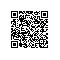# C++11 lambda表达式

C++11引入了lambda表达式，使得程序员可以定义匿名函数，该函数是一次性执行的，既方便了编程，又能防止别人的访问。

Lambda表达式的语法通过下图来介绍：这里假设我们定义了一个如上图的lambda表达式。现在来介绍途中标有编号的各个部分是什么意思。

1. Lambda表达式的引入标志，在‘[]’里面可以填入‘=’或‘&’表示该lambda表达式“捕获”（lambda表达式在一定的scope可以访问的数据）的数据时以什么方式捕获的，‘&’表示一引用的方式；‘=’表明以值传递的方式捕获，除非专门指出。
2. Lambda表达式的参数列表
3. Mutable 标识
4. 异常标识
5. 返回值
6. “函数”体，也就是lambda表达式需要进行的实际操作

int x = 10;

int y = 3;

int z ;

z = [=]()mutable throw() -> int { int n = x + y; x = y ; y = n; return n;}();

cout<<z<<endl;

cout<<"x:"<<x<<"\t"<<"y:"<<y<<endl;

13

x: 10  y: 3

#include <iostream>

using namespace std;

int main()

{

int n = [] (int x, int y) { return x + y; }(5, 4);

cout << n << endl;

}

#include <iostream>

using namespace std;

int main()

{

auto f = [] (int x, int y) { return x + y; };

cout << f(21, 12) << endl;

}

Lambda表达式与STL算法一起使用，自己写测试代码的时候经常用到排序、输出数组什么的，通过下面列举的几个算法也比较方便：

#include <iostream>

#include <algorithm>

#include <ctime>

using namespace std;

int main()

{

int a = {0};

srand(time(NULL));

generate(a,a+10,[]()->int { return rand() % 100; });

cout<<"before sort: "<<endl;

for_each(a, a+10, [&](int i){ cout<< i <<" "; });

cout<<endl;

cout<<"After sort"<<endl;

sort(a,a+10);

for_each(a, a+10, [&](int i){ cout<< i <<" "; });

return 0;

}

Lambda表达式的嵌套：

#include <iostream>

int main()

{

using namespace std;

int m = [](int x)

{ return [](int y) { return y * 2; }(x) + 3; }(5);

cout << m << endl;

}使用钉钉扫一扫加入圈子
+ 订阅